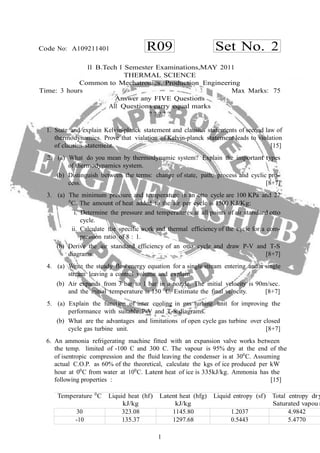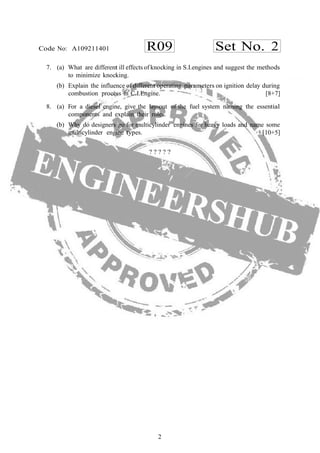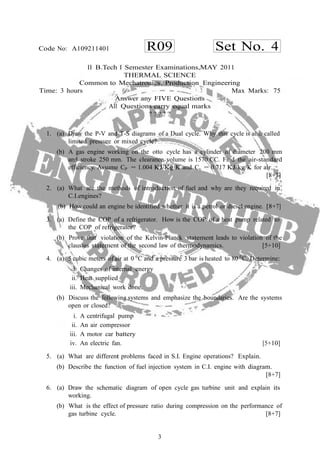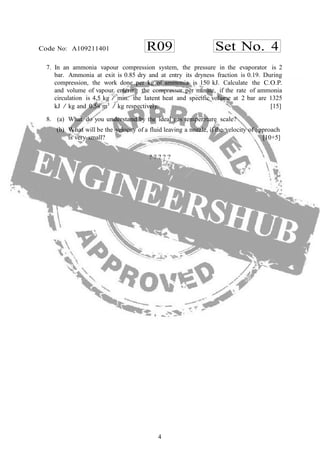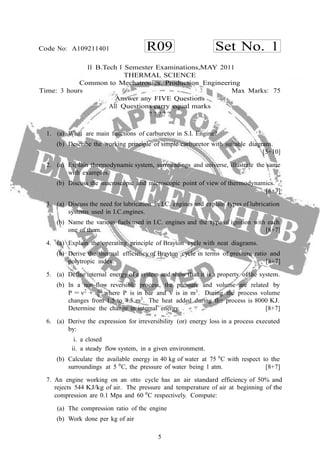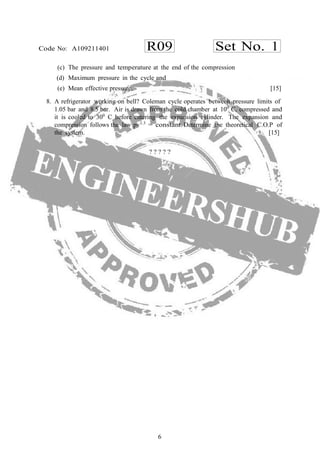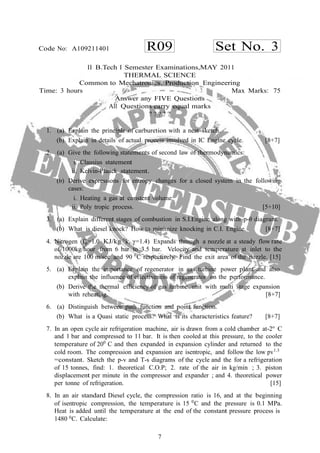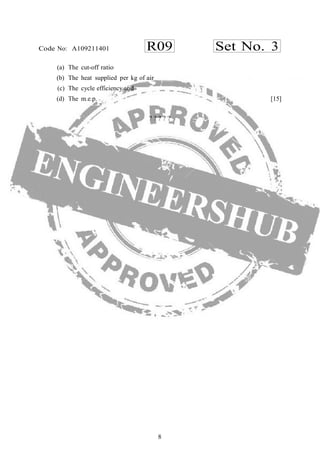Próximo SlideShare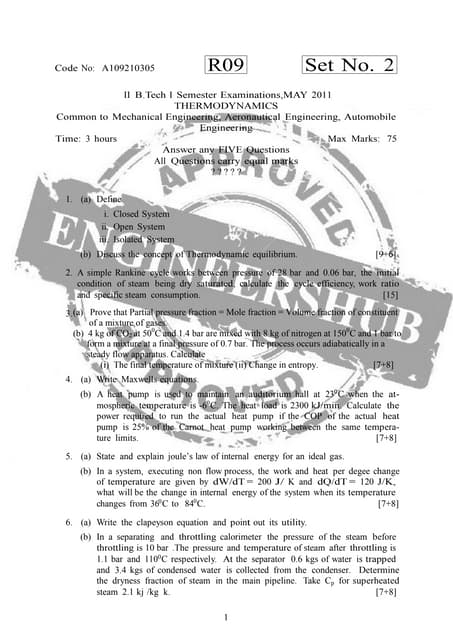A109210305 thermodynamics1
Cargando en ... 3
1 de 8A109210305 thermodynamics1jntuworldS.e 2003swapnil shindeMe2202 engineering thermodynamics uq - april may 2010BIBIN CHIDAMBARANATHANMe2202 engineering thermodynamics uq - april may 2011BIBIN CHIDAMBARANATHANMe2202 engineering thermodynamics uq - april may 2011BIBIN CHIDAMBARANATHANMe1201 engineering thermodynamics uq - may june 2007BIBIN CHIDAMBARANATHAN

### A109211401 thermalscience1

1. R09 Set No. 2Code No: A109211401 1 Temperature 0 C Liquid heat (hf) kJ/kg Latent heat (hfg) kJ/kg Liquid entropy (sf) Total entropy dr Saturated vapou 30 323.08 1145.80 1.2037 4.9842 -10 135.37 1297.68 0.5443 5.4770 II B.Tech I Semester Examinations,MAY 2011 THERMAL SCIENCE Common to Mechatronics, Production Engineering Time: 3 hours Max Marks: 75 Answer any FIVE Questions All Questions carry equal marks ? ? ? ? ? 1. State and explain Kelvin-planck statement and clausius statements of second law of thermodynamics. Prove that vialation of Kelvin-planck statement leads to violation of clausius statement.  2. (a) What do you mean by thermodynamic system? Explain the important types of thermodynamics system. (b) Distinguish between the terms: change of state, path, process and cyclic pro- cess. [8+7] 3. (a) The minimum pressure and temperature in an otto cycle are 100 KPa and 27 0 C. The amount of heat added to the air per cycle is 1500 KJ/Kg: i. Determine the pressure and temperatures at all points of air standard otto cycle. ii. Calculate the specific work and thermal efficiency of the cycle for a com- pression ratio of 8 : 1. (b) Derive the air standard efficiency of an otto cycle and draw P-V and T-S diagrams. [8+7] 4. (a) Write the steady flowenergy equation for a single stream entering and a single stream leaving a control volume and explain. (b) Air expands from 3 bar to 1 bar in a nozzle. The initial velocity is 90m/sec. and the initial temperature is 150 0 C. Estimate the final velocity. [8+7] 5. (a) Explain the function of inter cooling in gas turbine unit for improving the performance with suitable P-V and T-S diagrams. (b) What are the advantages and limitations of open cycle gas turbine over closed cycle gas turbine unit. [8+7] 6. An ammonia refrigerating machine fitted with an expansion valve works between the temp. limited of -100 C and 300 C. The vapour is 95% dry at the end of the of isentropic compression and the fluid leaving the condenser is at 300 C. Assuming actual C.O.P. as 60% of the theoretical, calculate the kgs of ice produced per kW hour at 00 C from water at 100 C. Latent heat of ice is 335kJ/kg. Ammonia has the following properties :  y r
2. R09 Set No. 2Code No: A109211401 2 7. (a) What are different ill effects of knocking in S.I.engines and suggest the methods to minimize knocking. (b) Explain the influence of different operating parameters on ignition delay during combustion process in C.I.Engine. [8+7] 8. (a) For a diesel engine, give the lay-out of the fuel system naming the essential components and explain their roles. (b) Why do designers go for multicylinder engines for heavy loads and name some multicylinder engine types. [10+5] ? ? ? ? ?
3. 3 R09 Set No. 4Code No: A109211401 II B.Tech I Semester Examinations,MAY 2011 THERMAL SCIENCE Common to Mechatronics, Production Engineering Time: 3 hours Max Marks: 75 Answer any FIVE Questions All Questions carry equal marks ? ? ? ? ? 1. (a) Draw the P-V and T-S diagrams of a Dual cycle. Why this cycle is also called limited pressure or mixed cycle? (b) A gas engine working on the otto cycle has a cylinder of diameter 200 mm and stroke 250 mm. The clearance volume is 1570 CC. Find the air-standard efficiency. Assume CP = 1.004 KJ/Kg K and Cv = 0.717 KJ/kg K for air. [8+7] 2. (a) What are the methods of introduction of fuel and why are they required in C.I.engines? (b) How could an engine be identified whether it is a petrol or diesel engine. [8+7] 3. (a) Define the COP of a refrigerator. How is the COP of a heat pump related to the COP of refrigerator? (b) Prove that violation of the Kelvin-Planck statement leads to violation of the clausius statement of the second law of thermodynamics. [5+10] 4. (a) 5 cubic meters of air at 0 0 C and a pressure 3 bar is heated to 80 0 C. Determine: i. Changes of internal energy ii. Heat supplied iii. Mechanical work done. (b) Discuss the following systems and emphasize the boundaries. Are the systems open or closed? i. A centrifugal pump ii. An air compressor iii. A motor car battery iv. An electric fan. [5+10] 5. (a) What are different problems faced in S.I. Engine operations? Explain. (b) Describe the function of fuel injection system in C.I. engine with diagram. [8+7] 6. (a) Draw the schematic diagram of open cycle gas turbine unit and explain its working. (b) What is the effect of pressure ratio during compression on the performance of gas turbine cycle. [8+7]
4. 4 R09 Set No. 4Code No: A109211401 7. In an ammonia vapour compression system, the pressure in the evaporator is 2 bar. Ammonia at exit is 0.85 dry and at entry its dryness fraction is 0.19. During compression, the work done per kg of ammonia is 150 kJ. Calculate the C.O.P. and volume of vapour entering the compressor per minute, if the rate of ammonia circulation is 4,5 kg / min. the latent heat and specific volume at 2 bar are 1325 kJ / kg and 0.58 m3 / kg respectively.  8. (a) What do you understand by the ideal gas temperature scale? (b) What will be the velocity of a fluid leaving a nozzle, if the velocity of approach is very small? [10+5] ? ? ? ? ?
5. 5 R09 Set No. 1Code No: A109211401 V II B.Tech I Semester Examinations,MAY 2011 THERMAL SCIENCE Common to Mechatronics, Production Engineering Time: 3 hours Max Marks: 75 Answer any FIVE Questions All Questions carry equal marks ? ? ? ? ? 1. (a) What are main functions of carburetor in S.I. Engine? (b) Describe the working principle of simple carburetor with suitable diagram. [5+10] 2. (a) Explain thermodynamic system, surroundings and universe, illustrate the same with examples. (b) Discuss the macroscopic and microscopic point of view of thermodynamics. [8+7] 3. (a) Discuss the need for lubrication in I.C. engines and explain types of lubrication systems used in I.C.engines. (b) Name the various fuels used in I.C. engines and the type of ignition with each one of them. [8+7] 4. (a) Explain the operating principle of Brayton cycle with neat diagrams. (b) Derive the thermal efficiency of Brayton cycle in terms of pressure ratio and polytropic index. [8+7] 5. (a) Define internal energy of a system and show that it is a property of the system. (b) In a non-flow reversible process, the pressure and volume are related by P = v2 + 10 where P is in bar and v is in m3 . During the process volume changes from 1.5 to 4.5 m3 . The heat added during the process is 8000 KJ. Determine the change in internal energy. [8+7] 6. (a) Derive the expression for irreversibility (or) energy loss in a process executed by: i. a closed ii. a steady flow system, in a given environment. (b) Calculate the available energy in 40 kg of water at 75 0 C with respect to the surroundings at 5 0 C, the pressure of water being 1 atm. [8+7] 7. An engine working on an otto cycle has an air standard efficiency of 50% and rejects 544 KJ/kg of air. The pressure and temperature of air at beginning of the compression are 0.1 Mpa and 60 0 C respectively. Compute: (a) The compression ratio of the engine (b) Work done per kg of air
6. 6 R09 Set No. 1Code No: A109211401 (c) The pressure and temperature at the end of the compression (d) Maximum pressure in the cycle and (e) Mean effective pressure.  8. A refrigerator working on bell? Coleman cycle operates between pressure limits of 1.05 bar and 8.5 bar. Air is drawn from the cold chamber at 100 C, compressed and it is cooled to 300 C before entering the expansion cylinder. The expansion and compression follows the law pv1.3 = constant. Determine the theoretical C.O.P of the system.  ? ? ? ? ?
7. 7 R09 Set No. 3Code No: A109211401 II B.Tech I Semester Examinations,MAY 2011 THERMAL SCIENCE Common to Mechatronics, Production Engineering Time: 3 hours Max Marks: 75 Answer any FIVE Questions All Questions carry equal marks ? ? ? ? ? 1. (a) Explain the principle of carburetion with a neat sketch. (b) Explain in details of actual process involved in IC Engine cycle. [8+7] 2. (a) Give the following statements of second law of thermodynamics: i. Clausius statement ii. Kelvin-Planck statement. (b) Derive expressions for entropy changes for a closed system in the following cases: i. Heating a gas at constent volume ii. Poly tropic process. [5+10] 3. (a) Explain different stages of combustion in S.I.Engine along with p-θ diagram. (b) What is diesel knock? How to minimize knocking in C.I. Engine. [8+7] 4. Nitrogen (Cp=1.0 KJ/kg 0 k, γ=1.4) Expands through a nozzle at a steady flow rate of 1000kg/hour from 6 bar to 3.5 bar. Velocity and temperature at inlet to the nozzle are 100 m/sec and 90 0 C respectively. Find the exit area of the nozzle.  5. (a) Explain the importance of regenerator in gas turbine power plant and also explain the influence of effectiveness of regenerator on the performance. (b) Derive the thermal efficiency of gas turbine unit with multi stage expansion with reheating. [8+7] 6. (a) Distinguish between path function and point function. (b) What is a Quasi static process? What is its characteristics feature? [8+7] 7. In an open cycle air refrigeration machine, air is drawn from a cold chamber at-2o C and 1 bar and compressed to 11 bar. It is then cooled at this pressure, to the cooler temperature of 200 C and then expanded in expansion cylinder and returned to the cold room. The compression and expansion are isentropic, and follow the low pv1.3 =constant. Sketch the p-v and T-s diagrams of the cycle and the for a refrigeration of 15 tonnes, find: 1. theoretical C.O.P; 2. rate of the air in kg/min ; 3. piston displacement per minute in the compressor and expander ; and 4. theoretical power per tonne of refrigeration.  8. In an air standard Diesel cycle, the compression ratio is 16, and at the beginning of isentropic compression, the temperature is 15 0 C and the pressure is 0.1 MPa. Heat is added until the temperature at the end of the constant pressure process is 1480 0C. Calculate:
8. 8 R09 Set No. 3Code No: A109211401 (a) The cut-off ratio (b) The heat supplied per kg of air (c) The cycle efficiency and (d) The m.e.p.  ? ? ? ? ?